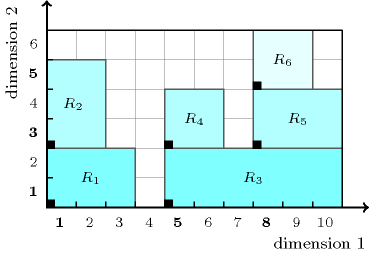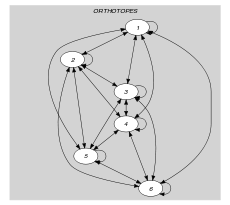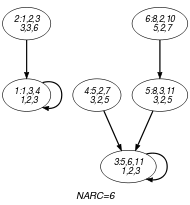## 5.323. place_in_pyramid

Origin

N. Beldiceanu

Constraint

$\mathrm{𝚙𝚕𝚊𝚌𝚎}_\mathrm{𝚒𝚗}_\mathrm{𝚙𝚢𝚛𝚊𝚖𝚒𝚍}\left(\mathrm{𝙾𝚁𝚃𝙷𝙾𝚃𝙾𝙿𝙴𝚂},\mathrm{𝚅𝙴𝚁𝚃𝙸𝙲𝙰𝙻}_\mathrm{𝙳𝙸𝙼}\right)$

Type
 $\mathrm{𝙾𝚁𝚃𝙷𝙾𝚃𝙾𝙿𝙴}$ $\mathrm{𝚌𝚘𝚕𝚕𝚎𝚌𝚝𝚒𝚘𝚗}\left(\mathrm{𝚘𝚛𝚒}-\mathrm{𝚍𝚟𝚊𝚛},\mathrm{𝚜𝚒𝚣}-\mathrm{𝚍𝚟𝚊𝚛},\mathrm{𝚎𝚗𝚍}-\mathrm{𝚍𝚟𝚊𝚛}\right)$
Arguments
 $\mathrm{𝙾𝚁𝚃𝙷𝙾𝚃𝙾𝙿𝙴𝚂}$ $\mathrm{𝚌𝚘𝚕𝚕𝚎𝚌𝚝𝚒𝚘𝚗}\left(\mathrm{𝚘𝚛𝚝𝚑}-\mathrm{𝙾𝚁𝚃𝙷𝙾𝚃𝙾𝙿𝙴}\right)$ $\mathrm{𝚅𝙴𝚁𝚃𝙸𝙲𝙰𝙻}_\mathrm{𝙳𝙸𝙼}$ $\mathrm{𝚒𝚗𝚝}$
Restrictions
 $|\mathrm{𝙾𝚁𝚃𝙷𝙾𝚃𝙾𝙿𝙴}|>0$ $\mathrm{𝚛𝚎𝚚𝚞𝚒𝚛𝚎}_\mathrm{𝚊𝚝}_\mathrm{𝚕𝚎𝚊𝚜𝚝}$$\left(2,\mathrm{𝙾𝚁𝚃𝙷𝙾𝚃𝙾𝙿𝙴},\left[\mathrm{𝚘𝚛𝚒},\mathrm{𝚜𝚒𝚣},\mathrm{𝚎𝚗𝚍}\right]\right)$ $\mathrm{𝙾𝚁𝚃𝙷𝙾𝚃𝙾𝙿𝙴}.\mathrm{𝚜𝚒𝚣}\ge 0$ $\mathrm{𝙾𝚁𝚃𝙷𝙾𝚃𝙾𝙿𝙴}.\mathrm{𝚘𝚛𝚒}\le \mathrm{𝙾𝚁𝚃𝙷𝙾𝚃𝙾𝙿𝙴}.\mathrm{𝚎𝚗𝚍}$ $\mathrm{𝚛𝚎𝚚𝚞𝚒𝚛𝚎𝚍}$$\left(\mathrm{𝙾𝚁𝚃𝙷𝙾𝚃𝙾𝙿𝙴𝚂},\mathrm{𝚘𝚛𝚝𝚑}\right)$ $\mathrm{𝚜𝚊𝚖𝚎}_\mathrm{𝚜𝚒𝚣𝚎}$$\left(\mathrm{𝙾𝚁𝚃𝙷𝙾𝚃𝙾𝙿𝙴𝚂},\mathrm{𝚘𝚛𝚝𝚑}\right)$ $\mathrm{𝚅𝙴𝚁𝚃𝙸𝙲𝙰𝙻}_\mathrm{𝙳𝙸𝙼}\ge 1$ $\mathrm{𝚍𝚒𝚏𝚏𝚗}$$\left(\mathrm{𝙾𝚁𝚃𝙷𝙾𝚃𝙾𝙿𝙴𝚂}\right)$
Purpose

For each pair of orthotopes $\left({O}_{1},{O}_{2}\right)$ of the collection $\mathrm{𝙾𝚁𝚃𝙷𝙾𝚃𝙾𝙿𝙴𝚂}$, ${O}_{1}$ and ${O}_{2}$ do not overlap (two orthotopes do not overlap if there exists at least one dimension where their projections do not overlap). In addition, each orthotope of the collection $\mathrm{𝙾𝚁𝚃𝙷𝙾𝚃𝙾𝙿𝙴𝚂}$ should be supported by one other orthotope or by the ground. The vertical dimension is given by the parameter $\mathrm{𝚅𝙴𝚁𝚃𝙸𝙲𝙰𝙻}_\mathrm{𝙳𝙸𝙼}$.

Example
$\left(\begin{array}{c}〈\begin{array}{c}\mathrm{𝚘𝚛𝚝𝚑}-〈\mathrm{𝚘𝚛𝚒}-1\mathrm{𝚜𝚒𝚣}-3\mathrm{𝚎𝚗𝚍}-4,\mathrm{𝚘𝚛𝚒}-1\mathrm{𝚜𝚒𝚣}-2\mathrm{𝚎𝚗𝚍}-3〉,\hfill \\ \mathrm{𝚘𝚛𝚝𝚑}-〈\mathrm{𝚘𝚛𝚒}-1\mathrm{𝚜𝚒𝚣}-2\mathrm{𝚎𝚗𝚍}-3,\mathrm{𝚘𝚛𝚒}-3\mathrm{𝚜𝚒𝚣}-3\mathrm{𝚎𝚗𝚍}-6〉,\hfill \\ \mathrm{𝚘𝚛𝚝𝚑}-〈\begin{array}{ccc}\mathrm{𝚘𝚛𝚒}-5\hfill & \mathrm{𝚜𝚒𝚣}-6\hfill & \mathrm{𝚎𝚗𝚍}-11,\hfill \\ \mathrm{𝚘𝚛𝚒}-1\hfill & \mathrm{𝚜𝚒𝚣}-2\hfill & \mathrm{𝚎𝚗𝚍}-3\hfill \end{array}〉,\hfill \\ \mathrm{𝚘𝚛𝚝𝚑}-〈\mathrm{𝚘𝚛𝚒}-5\mathrm{𝚜𝚒𝚣}-2\mathrm{𝚎𝚗𝚍}-7,\mathrm{𝚘𝚛𝚒}-3\mathrm{𝚜𝚒𝚣}-2\mathrm{𝚎𝚗𝚍}-5〉,\hfill \\ \mathrm{𝚘𝚛𝚝𝚑}-〈\begin{array}{ccc}\mathrm{𝚘𝚛𝚒}-8\hfill & \mathrm{𝚜𝚒𝚣}-3\hfill & \mathrm{𝚎𝚗𝚍}-11,\hfill \\ \mathrm{𝚘𝚛𝚒}-3\hfill & \mathrm{𝚜𝚒𝚣}-2\hfill & \mathrm{𝚎𝚗𝚍}-5\hfill \end{array}〉,\hfill \\ \mathrm{𝚘𝚛𝚝𝚑}-〈\begin{array}{ccc}\mathrm{𝚘𝚛𝚒}-8\hfill & \mathrm{𝚜𝚒𝚣}-2\hfill & \mathrm{𝚎𝚗𝚍}-10,\hfill \\ \mathrm{𝚘𝚛𝚒}-5\hfill & \mathrm{𝚜𝚒𝚣}-2\hfill & \mathrm{𝚎𝚗𝚍}-7\hfill \end{array}〉\hfill \end{array}〉,2\hfill \end{array}\right)$

Figure 5.323.1 depicts the placement associated with the example, where the ${i}^{th}$ item of the $\mathrm{𝙾𝚁𝚃𝙷𝙾𝚃𝙾𝙿𝙴𝚂}$ collection is represented by the rectangle Ri. The $\mathrm{𝚙𝚕𝚊𝚌𝚎}_\mathrm{𝚒𝚗}_\mathrm{𝚙𝚢𝚛𝚊𝚖𝚒𝚍}$ constraint holds since the rectangles do not overlap and since rectangles R1, R2, R3, R4, R5, and R6 are respectively supported by the ground, R1, the ground, R3, R3, and R5.

##### Figure 5.323.1. Solution corresponding to the Example slotTypical
 $|\mathrm{𝙾𝚁𝚃𝙷𝙾𝚃𝙾𝙿𝙴}|>1$ $\mathrm{𝙾𝚁𝚃𝙷𝙾𝚃𝙾𝙿𝙴}.\mathrm{𝚜𝚒𝚣}>0$ $|\mathrm{𝙾𝚁𝚃𝙷𝙾𝚃𝙾𝙿𝙴𝚂}|>1$
Symmetry

Items of $\mathrm{𝙾𝚁𝚃𝙷𝙾𝚃𝙾𝙿𝙴𝚂}$ are permutable.

Usage

The $\mathrm{𝚍𝚒𝚏𝚏𝚗}$ constraint is not enough if one wants to produce a placement where no orthotope floats in the air. This constraint is usually handled with a heuristic during the enumeration phase.

Keywords
Arc input(s)

$\mathrm{𝙾𝚁𝚃𝙷𝙾𝚃𝙾𝙿𝙴𝚂}$

Arc generator
$\mathrm{𝐶𝐿𝐼𝑄𝑈𝐸}$$↦\mathrm{𝚌𝚘𝚕𝚕𝚎𝚌𝚝𝚒𝚘𝚗}\left(\mathrm{𝚘𝚛𝚝𝚑𝚘𝚝𝚘𝚙𝚎𝚜}\mathtt{1},\mathrm{𝚘𝚛𝚝𝚑𝚘𝚝𝚘𝚙𝚎𝚜}\mathtt{2}\right)$

Arc arity
Arc constraint(s)
$\bigvee \left(\begin{array}{c}\bigwedge \left(\begin{array}{c}\mathrm{𝚘𝚛𝚝𝚑𝚘𝚝𝚘𝚙𝚎𝚜}\mathtt{1}.\mathrm{𝚔𝚎𝚢}=\mathrm{𝚘𝚛𝚝𝚑𝚘𝚝𝚘𝚙𝚎𝚜}\mathtt{2}.\mathrm{𝚔𝚎𝚢},\hfill \\ \mathrm{𝚘𝚛𝚝𝚑}_\mathrm{𝚘𝚗}_\mathrm{𝚝𝚑𝚎}_\mathrm{𝚐𝚛𝚘𝚞𝚗𝚍}\left(\mathrm{𝚘𝚛𝚝𝚑𝚘𝚝𝚘𝚙𝚎𝚜}\mathtt{1}.\mathrm{𝚘𝚛𝚝𝚑},\mathrm{𝚅𝙴𝚁𝚃𝙸𝙲𝙰𝙻}_\mathrm{𝙳𝙸𝙼}\right)\hfill \end{array}\right),\hfill \\ \bigwedge \left(\begin{array}{c}\mathrm{𝚘𝚛𝚝𝚑𝚘𝚝𝚘𝚙𝚎𝚜}\mathtt{1}.\mathrm{𝚔𝚎𝚢}\ne \mathrm{𝚘𝚛𝚝𝚑𝚘𝚝𝚘𝚙𝚎𝚜}\mathtt{2}.\mathrm{𝚔𝚎𝚢},\hfill \\ \mathrm{𝚘𝚛𝚝𝚑}_\mathrm{𝚘𝚗}_\mathrm{𝚝𝚘𝚙}_\mathrm{𝚘𝚏}_\mathrm{𝚘𝚛𝚝𝚑}\left(\begin{array}{c}\mathrm{𝚘𝚛𝚝𝚑𝚘𝚝𝚘𝚙𝚎𝚜}\mathtt{1}.\mathrm{𝚘𝚛𝚝𝚑},\hfill \\ \mathrm{𝚘𝚛𝚝𝚑𝚘𝚝𝚘𝚙𝚎𝚜}\mathtt{2}.\mathrm{𝚘𝚛𝚝𝚑},\hfill \\ \mathrm{𝚅𝙴𝚁𝚃𝙸𝙲𝙰𝙻}_\mathrm{𝙳𝙸𝙼}\hfill \end{array}\right)\hfill \end{array}\right)\hfill \end{array}\right)$
Graph property(ies)
$\mathrm{𝐍𝐀𝐑𝐂}$$=|\mathrm{𝙾𝚁𝚃𝙷𝙾𝚃𝙾𝙿𝙴𝚂}|$

Graph model

The arc constraint of the graph constraint forces one of the following conditions:

• If the arc connects the same orthotope $O$ then the ground directly supports $O$,

• Otherwise, if we have an arc from an orthotope ${O}_{1}$ to a distinct orthotope ${O}_{2}$, the condition is: ${O}_{1}$ is on top of ${O}_{2}$ (i.e., in all dimensions, except dimension $\mathrm{𝚅𝙴𝚁𝚃𝙸𝙲𝙰𝙻}_\mathrm{𝙳𝙸𝙼}$, the projection of ${O}_{1}$ is included in the projection of ${O}_{2}$, while in dimension $\mathrm{𝚅𝙴𝚁𝚃𝙸𝙲𝙰𝙻}_\mathrm{𝙳𝙸𝙼}$ the projection of ${O}_{1}$ is located after the projection of ${O}_{2}$).

Parts (A) and (B) of Figure 5.323.2 respectively show the initial and final graph associated with the Example slot. Since we use the $\mathrm{𝐍𝐀𝐑𝐂}$ graph property, the arcs of the final graph are stressed in bold.

##### Figure 5.323.2. Initial and final graph of the $\mathrm{𝚙𝚕𝚊𝚌𝚎}_\mathrm{𝚒𝚗}_\mathrm{𝚙𝚢𝚛𝚊𝚖𝚒𝚍}$ constraint(a) (b)